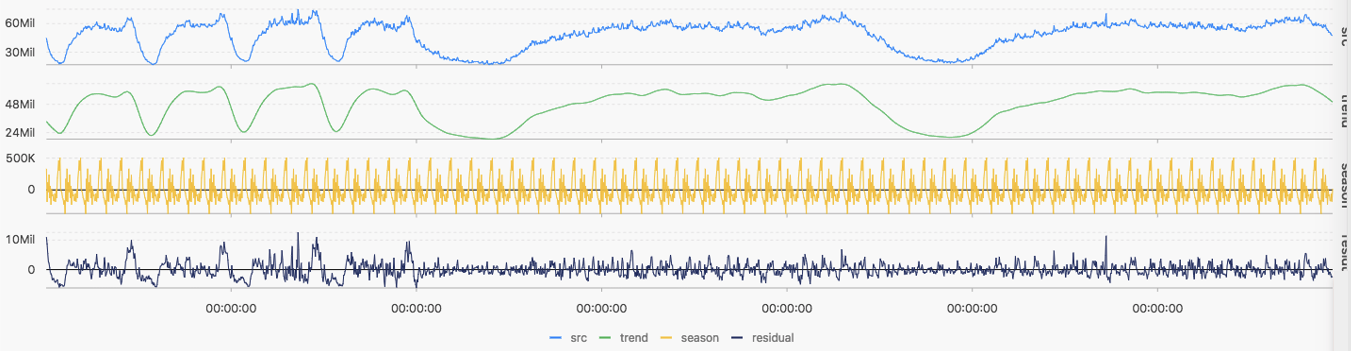The sequence decomposition function can decompose service curves and highlight information about the curve trends and periods.

## ts_decompose

Function format:
``select ts_decompose(x, y, samplePeriod, sampleMethod) ``
The following table describes the parameters.
Parameter Description Value
x Time column in ascending order Unixtime timestamp in seconds
y Numeric column corresponding to the data at a specified time point -
samplePeriod Period during which the current time series data is sampled Long type values ranging from 1 to 86399 seconds
sampleMethod Method for sampling the data in the sampling window Value range:
• avg: average value of the data in the window
• max: maximum value of the data in the window
• min: minimum value of the data in the window
• sum: sum of the data in the window
Example:
• Statement for query and analysis:
``* | select ts_decompose(stamp, value, 1, 'avg') from (select __time__ - __time__ % 60 as stamp, avg(v) as value from log GROUP BY stamp order by stamp)``
• Result:The following table describes the display items.
Display item Description
Horizontal axis unixtime Unixtime timestamp in seconds
Longitudinal axis src Raw data
trend Curve trend after decomposition
season Curve period after decomposition
residual Residual data after decomposition